# RD Sharma Solutions For Class 7 Maths Chapter 1 Integers Exercise 1.4

In order to excel in board exams, students can view and download the RD Sharma Solutions For Class 7 Maths Exercise 1.4 Chapter 1 PDF from the given links. Students who find it challenging to solve complex Math problems can refer to the RD Sharma Solutions for better guidance. Solving this exercise using the solutions PDF will ensure that students excel in board exams. In this exercise, we shall study the use of brackets. By practising the RD Sharma Solutions for Class 7, students will be thorough in the concepts.

## Download the PDF of RD Sharma Solutions For Class 7 Maths Chapter 1 – Integers Exercise 1.4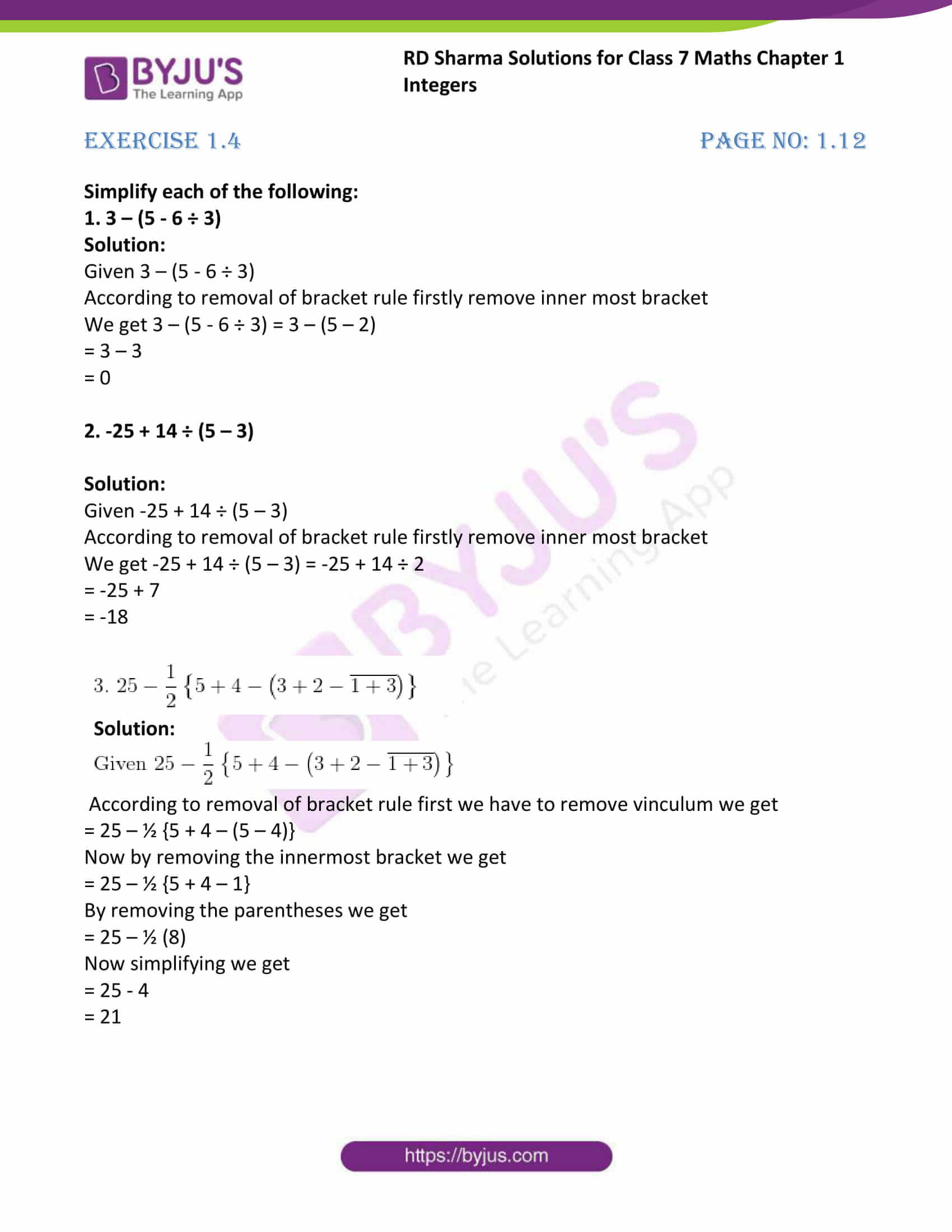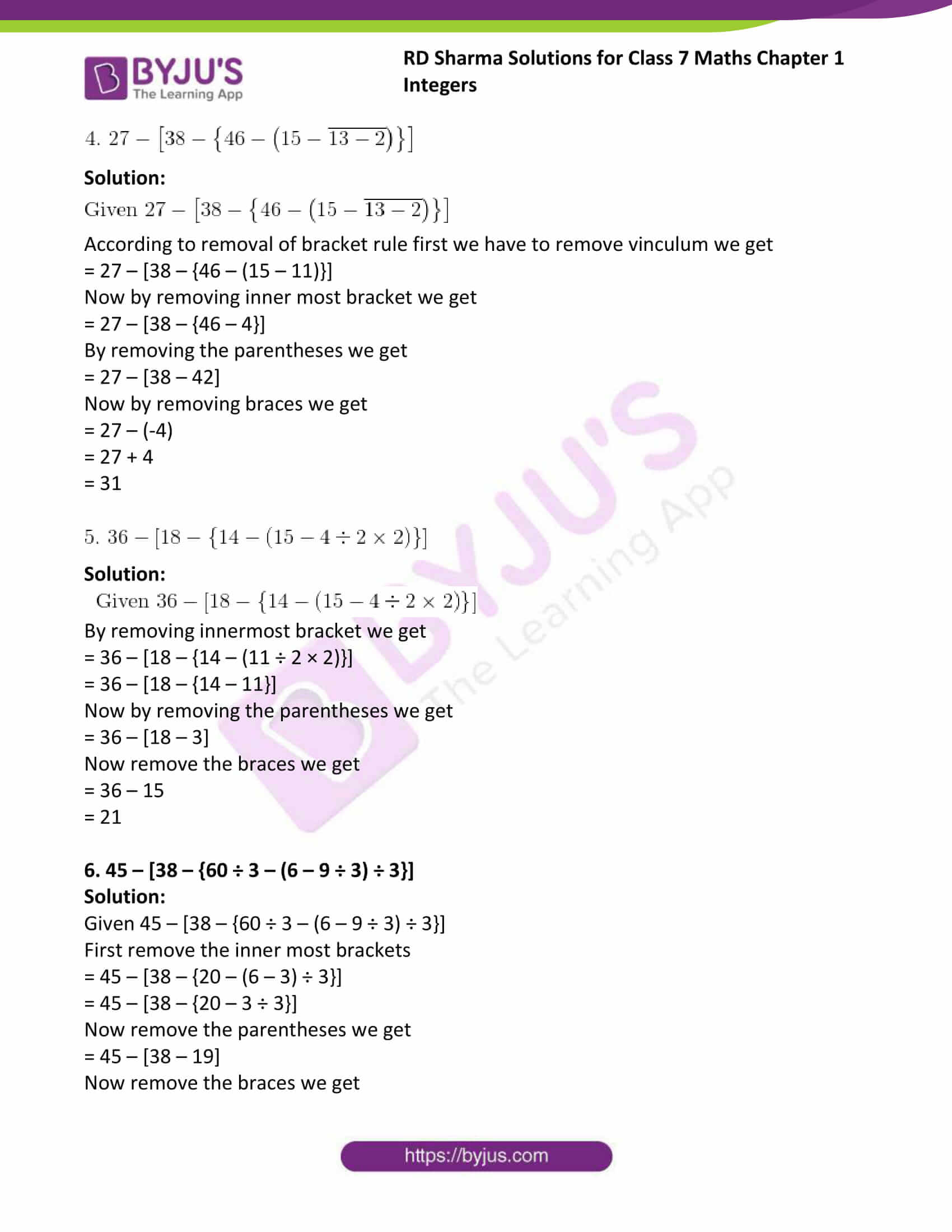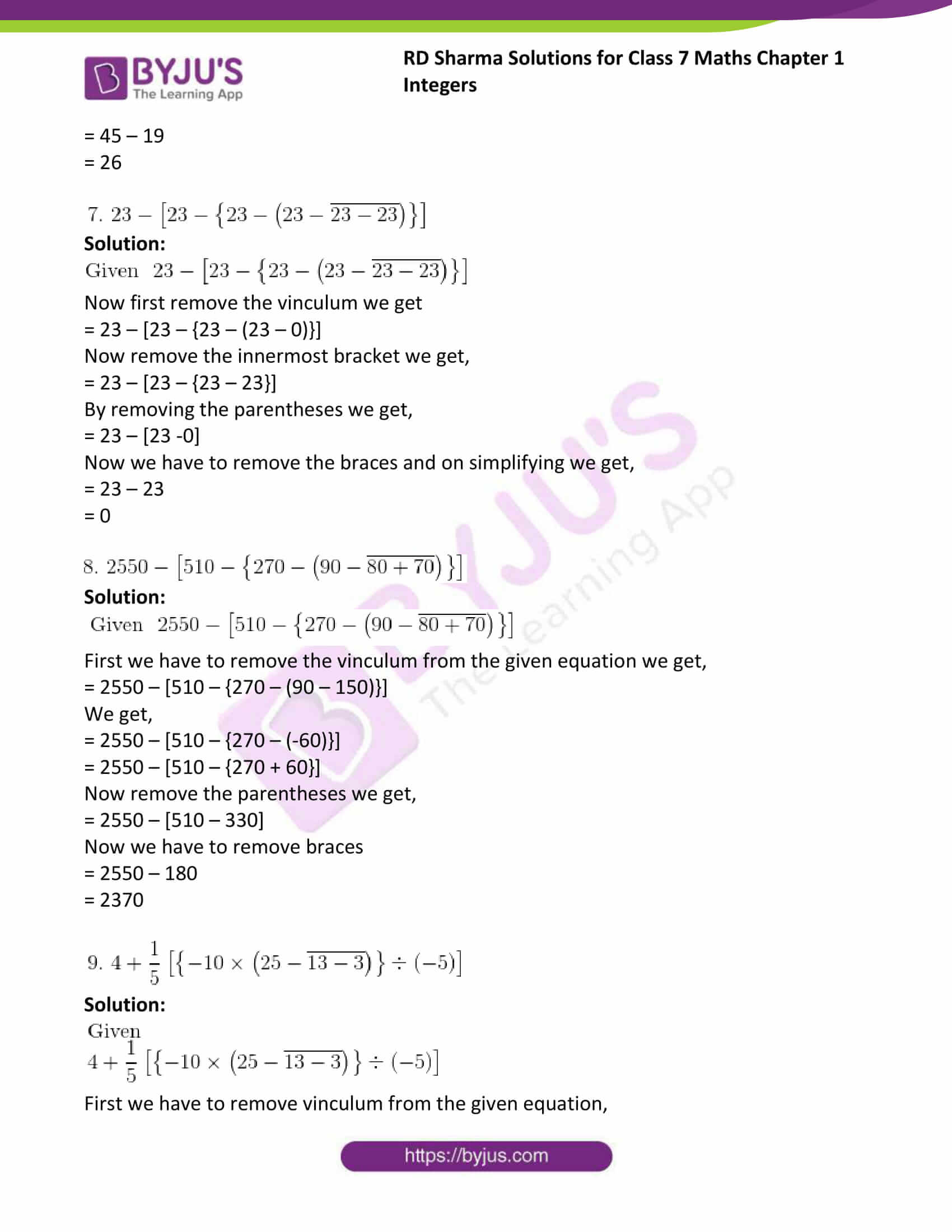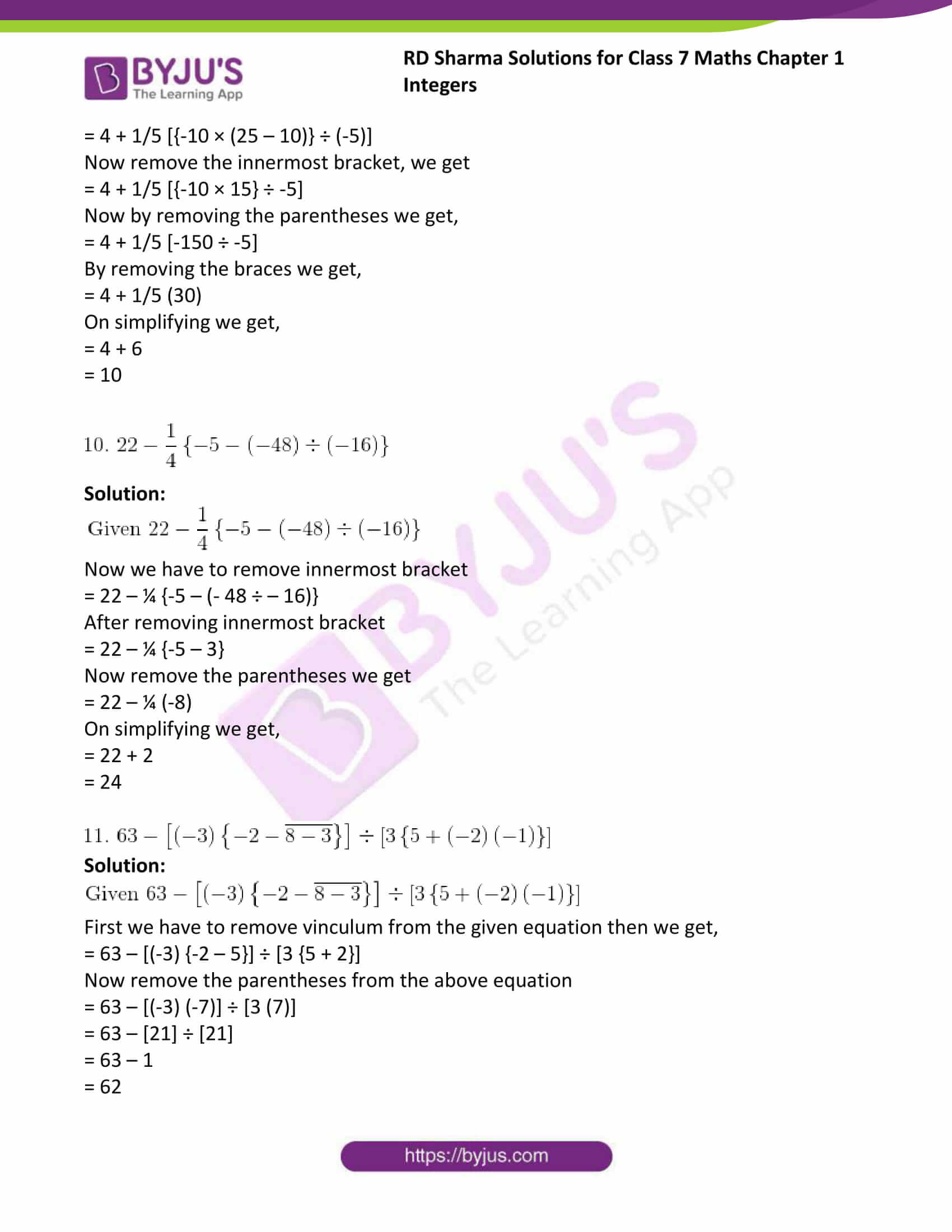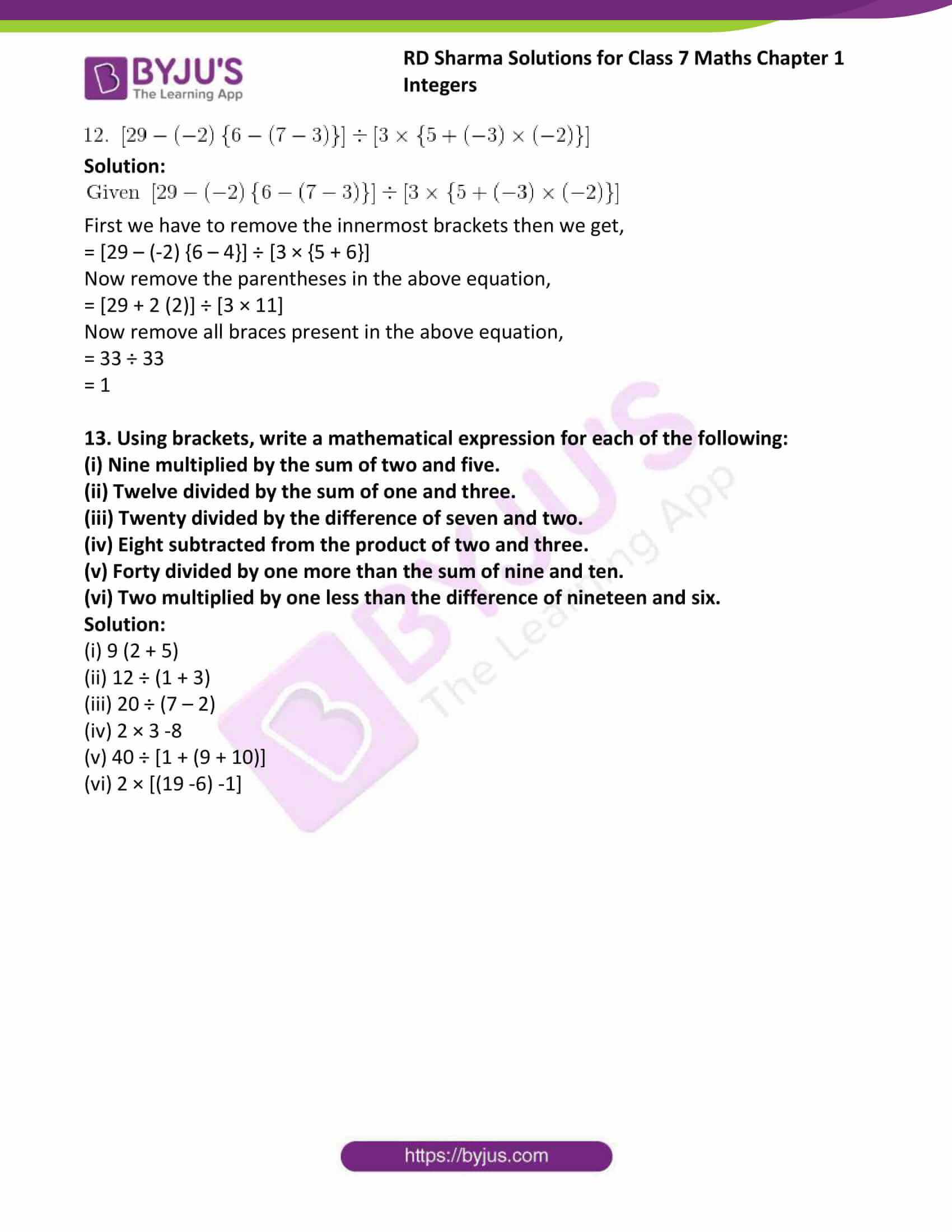### Access answers to Maths RD Sharma Solutions For Class 7 Chapter 1 – Integers Exercise 1.4

Exercise 1.4 Page No: 1.12

Simplify each of the following:

1. 3 – (5 – 6 ÷ 3)

Solution:

Given 3 – (5 – 6 ÷ 3)

According to removal of bracket rule firstly remove inner most bracket

We get 3 – (5 – 6 ÷ 3) = 3 – (5 – 2)

= 3 – 3

= 0

2. -25 + 14 ÷ (5 – 3)

Solution:

Given -25 + 14 ÷ (5 – 3)

According to removal of bracket rule firstly remove inner most bracket

We get -25 + 14 ÷ (5 – 3) = -25 + 14 ÷ 2

= -25 + 7

= -18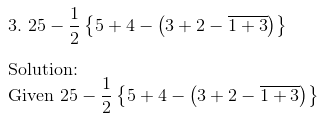Solution:According to removal of bracket rule first we have to remove vinculum we get

= 25 – ½ {5 + 4 – (5 – 4)}

Now by removing the innermost bracket we get

= 25 – ½ {5 + 4 – 1}

By removing the parentheses we get

= 25 – ½ (8)

Now simplifying we get

= 25 – 4

= 21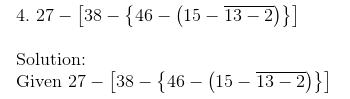Solution:According to removal of bracket rule first we have to remove vinculum we get

= 27 – [38 – {46 – (15 – 11)}]

Now by removing inner most bracket we get

= 27 – [38 – {46 – 4}]

By removing the parentheses we get

= 27 – [38 – 42]

Now by removing braces we get

= 27 – (-4)

= 27 + 4

= 31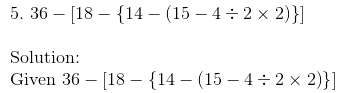Solution:By removing innermost bracket we get

= 36 – [18 – {14 – (11 ÷ 2 × 2)}]

= 36 – [18 – {14 – 11}]

Now by removing the parentheses we get

= 36 – [18 – 3]

Now remove the braces we get

= 36 – 15

= 21

6. 45 – [38 – {60 ÷ 3 – (6 – 9 ÷ 3) ÷ 3}]

Solution:

Given 45 – [38 – {60 ÷ 3 – (6 – 9 ÷ 3) ÷ 3}]

First remove the inner most brackets

= 45 – [38 – {20 – (6 – 3) ÷ 3}]

= 45 – [38 – {20 – 3 ÷ 3}]

Now remove the parentheses we get

= 45 – [38 – 19]

Now remove the braces we get

= 45 – 19

= 26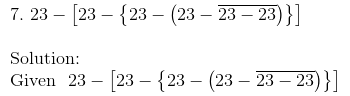Solution:Now first remove the vinculum we get

= 23 – [23 – {23 – (23 – 0)}]

Now remove the innermost bracket we get,

= 23 – [23 – {23 – 23}]

By removing the parentheses we get,

= 23 – [23 -0]

Now we have to remove the braces and on simplifying we get,

= 23 – 23

= 0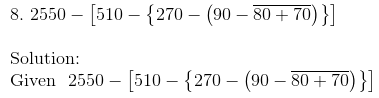Solution:First we have to remove the vinculum from the given equation we get,

= 2550 – [510 – {270 – (90 – 150)}]

We get,

= 2550 – [510 – {270 – (-60)}]

= 2550 – [510 – {270 + 60}]

Now remove the parentheses we get,

= 2550 – [510 – 330]

Now we have to remove braces

= 2550 – 180

= 2370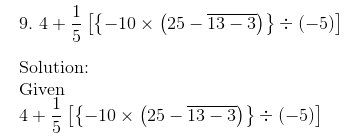Solution:First we have to remove vinculum from the given equation,

= 4 + 1/5 [{-10 × (25 – 10)} ÷ (-5)]

Now remove the innermost bracket, we get

= 4 + 1/5 [{-10 × 15} ÷ -5]

Now by removing the parentheses we get,

= 4 + 1/5 [-150 ÷ -5]

By removing the braces we get,

= 4 + 1/5 (30)

On simplifying we get,

= 4 + 6

= 10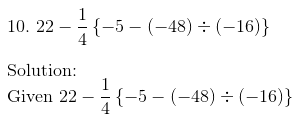Solution:Now we have to remove innermost bracket

= 22 – ¼ {-5 – (- 48 ÷ – 16)}

After removing innermost bracket

= 22 – ¼ {-5 – 3}

Now remove the parentheses we get

= 22 – ¼ (-8)

On simplifying we get,

= 22 + 2

= 24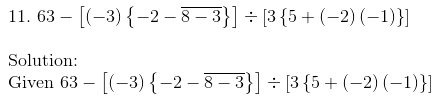Solution:First we have to remove vinculum from the given equation then we get,

= 63 – [(-3) {-2 – 5}] ÷ [3 {5 + 2}]

Now remove the parentheses from the above equation

= 63 – [(-3) (-7)] ÷ [3 (7)]

= 63 –  ÷ 

= 63 – 1

= 62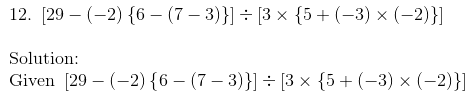Solution:First we have to remove the innermost brackets then we get,

= [29 – (-2) {6 – 4}] ÷ [3 × {5 + 6}]

Now remove the parentheses in the above equation,

= [29 + 2 (2)] ÷ [3 × 11]

Now remove all braces present in the above equation,

= 33 ÷ 33

= 1

13. Using brackets, write a mathematical expression for each of the following:

(i) Nine multiplied by the sum of two and five.

(ii) Twelve divided by the sum of one and three.

(iii) Twenty divided by the difference of seven and two.

(iv) Eight subtracted from the product of two and three.

(v) Forty divided by one more than the sum of nine and ten.

(vi) Two multiplied by one less than the difference of nineteen and six.

Solution:

(i) 9 (2 + 5)

(ii) 12 ÷ (1 + 3)

(iii) 20 ÷ (7 – 2)

(iv) 2 × 3 -8

(v) 40 ÷ [1 + (9 + 10)]

(vi) 2 × [(19 -6) -1]

## RD Sharma Solutions for Class 7 Maths Chapter – 1 Integers Exercise 1.4

Exercise 1.4 of RD Sharma Solutions for Class 7 Maths Chapter 1 Integers deals with the use of brackets. Sometimes in complex expressions, we require a set of operations to be performed prior to the others; the use of brackets explains this. To simplify expressions involving more than one bracket, we use the removal of brackets rule. Hence, the preparation of exams using the RD Sharma Solutions will definitely provide students with the advantage of scoring well.